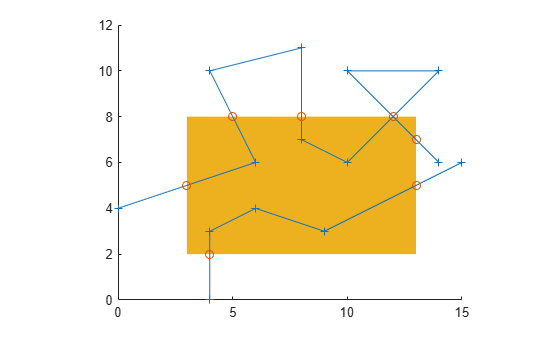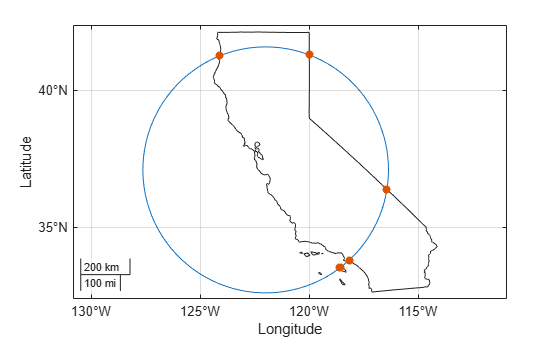# polyxpoly

Intersection points for lines or polygon edges

## Syntax

``[xi,yi] = polyxpoly(x1,y1,x2,y2)``
``[xi,yi,ii] = polyxpoly(___)``
``[xi,yi] = polyxpoly(___,'unique')``

## Description

example

````[xi,yi] = polyxpoly(x1,y1,x2,y2)` returns the intersection points of two polylines in a planar, Cartesian system, with vertices defined by `x1`, `y1`, `x2` and `y2`. The output arguments, `xi` and `yi`, contain the x- and y-coordinates of each point at which a segment of the first polyline intersects a segment of the second. In the case of overlapping, collinear segments, the intersection is actually a line segment rather than a point, and both endpoints are included in `xi`, `yi`.```
````[xi,yi,ii] = polyxpoly(___)` returns a two-column array of line segment indices corresponding to the intersection points. The k-th row of `ii` indicates which polyline segments give rise to the intersection point `xi(k)`, `yi(k)`.To remember how these indices work, just think of segments and vertices as fence sections and posts. The i-th fence section connects the i-th post to the (i+1)-th post. In general, letting i and j denote the scalar values comprised by the k-th row of `ii`, the intersection indicated by that row occurs where the i-th segment of the first polyline intersects the j-th segment of the second polyline. But when an intersection falls precisely on a vertex of the first polyline, then i is the index of that vertex. Likewise with the second polyline and the index j. In the case of an intersection at the i-th vertex of the first line, for example, `xi(k)` equals `x1(i)` and `yi(k)` equals `y1(i)`. In the case of intersections between vertices, i and j can be interpreted as follows: the segment connecting `x1(i)`, `y1(i)` to `x1(i+1)`, `y1(i+1)` intersects the segment connecting `x2(j)`, `y2(j)` to `x2(j+1)`, `y2(j+1)` at the point `xi(k)`, `yi(k)`.```

example

````[xi,yi] = polyxpoly(___,'unique')` filters out duplicate intersections, which may result if the input polylines are self-intersecting.```

## Examples

collapse all

Define and fill a rectangular area in the plane.

```xlimit = [3 13]; ylimit = [2 8]; xbox = xlimit([1 1 2 2 1]); ybox = ylimit([1 2 2 1 1]); mapshow(xbox,ybox,'DisplayType','polygon','LineStyle','none')```

Define and display a two-part polyline.

```x = [0 6 4 8 8 10 14 10 14 NaN 4 4 6 9 15]; y = [4 6 10 11 7 6 10 10 6 NaN 0 3 4 3 6]; mapshow(x,y,'Marker','+')```

Intersect the polyline with the rectangle.

```[xi,yi] = polyxpoly(x,y,xbox,ybox); mapshow(xi,yi,'DisplayType','point','Marker','o')```Display the intersection points; note that the point (12, 8) appears twice because of a self-intersection near the end of the first part of the polyline.

`[xi yi]`
```ans = 8×2 3.0000 5.0000 5.0000 8.0000 8.0000 8.0000 12.0000 8.0000 12.0000 8.0000 13.0000 7.0000 13.0000 5.0000 4.0000 2.0000 ```

You can suppress this duplicate point by using the `'unique'` option.

```[xi,yi] = polyxpoly(x,y,xbox,ybox,'unique'); [xi yi]```
```ans = 7×2 3.0000 5.0000 5.0000 8.0000 8.0000 8.0000 12.0000 8.0000 13.0000 7.0000 13.0000 5.0000 4.0000 2.0000 ```

Read state polygons into a geospatial table. Create a subtable that contains the California polygon. Display the polygon on a map.

```states = readgeotable("usastatehi.shp"); row = states.Name == "California"; california = states(row,:); figure usamap("california") geoshow(california,"FaceColor","none")```

Define a small circle centered off the coast of California.

```lat0 = 37; lon0 = -122; rad = 500; [latc,lonc] = scircle1(lat0,lon0,km2deg(rad)); plotm(lat0,lon0,"r*") plotm(latc,lonc,"r")```

Extract the latitude and longitude coordinates of the California polygon from the geospatial table.

```T = geotable2table(california,["Latitude","Longitude"]); [lat,lon] = polyjoin(T.Latitude',T.Longitude');```

Find the intersection points between the state of California and the small circle.

```[loni,lati] = polyxpoly(lon,lat,lonc,latc); plotm(lati,loni,"bo")```## Input Arguments

collapse all

x- or y-coordinates of points in the first or second polyline, specified as a numeric vector. For a given polyline, the x- and y-coordinate vectors must be the same length.

## Output Arguments

collapse all

x- or y-coordinates of intersection points, specified as a numeric column vector.

Line segment indices of intersection points, specified as a numeric vector.

## Tips

• If the spacing between points is large, the intersections calculated by the `polyxpoly` function and the intersections shown on a map display might be different. This is a result of differences between straight lines in the unprojected and projected coordinates. Similarly, there might be differences between the `polyxpoly` result and intersections that assume great circles or rhumb lines between points.

## Version History

Introduced before R2006a